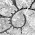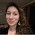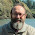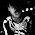## Friday, August 17, 2012

### Bayesian MCMC for the threshold model

I'm going to write a shorter post about this than it deserves, but I have just programmed & posted a simple Bayesian MCMC version of the threshold model for discrete character evolution. This is the same model that is described by Felsenstein (2012) in his recent Am. Nat. paper.

In the quantitative genetic threshold model, we have a discretely valued characteristic that has an underlying quantitative basis, called liability. When the liability exceeds some threshold value, the trait changes state (say, from absence to presence or vice versa). The appeal of this model for analyzing discrete character evolution in the context of the tree is that it is (for many traits) more biologically realistic than a Markov-process model in which characters spontaneously turn on and off; and the probability of turning back on is independent of the time since "off." In addition, it allows us a framework for estimating the correlations between discrete and continuous characters, or between multiple discrete characters - this is just the correlation of their liabilities. For more about this model, I recommend the reference above.

Joe has already implemented a version of this model in his stand-alone program Threshml. There are some important differences between his implementation and my Bayesian version in R (threshBayes). These differences mean that parameter estimation will be highly similar, but not the same, between the two methods:

1. In Threshml, Joe uses MCMC to sample the liabilities for tip and internal nodes on the tree, and then computes the MLEs of the Brownian variances & covariances conditioned on the sampled liabilities. In my program, the liabilities, ancestral states at the root, correlation, and Brownian rates are sampled from their joint posterior probability distribution.

2. Because threshBayes is Bayesian, the user can control the prior probability distributions for the different parameters in the model. (Although if these are not supplied the program will try and compute sensible priors.)

3. The function threshBayes outputs the posterior sample, rather than a summary of the parameter estimates. This leaves more for the user to do, but it also means that the user can evaluate convergence & the like using MCMC diagnostics (such as those in the package 'coda').

4. Threshml runs multiple chains and combines the post burn-in results, whereas threshBayes only runs one chain. This doesn't seem to be that important, but if users want to run multiple chains they can do so manually, of course.

5. Threshml samples the liabilities at internal and tip nodes. threshBayes samples only the tip liabilities, and then computes their probability based on the sampled parameter values of the evolutionary model and root states on the tree.

6. Finally, as of now, Threshml can analyze an arbitrary number of characters; whereas threshBayes can only accept two traits (which can be any combination of discrete & continuous traits).

Check out the code for threshBayes on my phytools page. After lunch, I'll give an example simulation & result.

1.Since you didn't mention this in the post, I want to point out that MCMCglmm package already has a Bayesian version of the threshold model implemented, I think.

2.Thanks for the comment.

This is certainly possible, of course. The issue is specifically addressed (but without a satisfying resolution) in Felsenstein (2012; p. 146) in the passage beginning "Hadfield and Nakagawa (2010) have noted that all such models are equivalent to multivariate “mixed models” of quantitative genetics. For discrete traits. . . ."

Do you know how you would design this test using the MCMCglmm package? Is this documented in the literature or on the web? A simple Google search of "threshold" with "MCMCglmm" doesn't turn up any useful results. How about natural extensions of this model to multiple ordered states and multiple states with an underlying (but unknown) order?

Thanks for the suggestion! Liam

3.After thinking about it, this may similar to fitting a phylogenetic glmm model with a logit link function though I am completely unsure about this. This code may be completely wrong but it runs.

require(MCMCglmm)
require(geiger)

# i am just going input a simulated tree of 100 taxa

tree <- drop.tip(birthdeath.tree(1, 0, taxa.stop=101), "101")

## simulate two completely uncorrelated traits just for an example

q <- list(rbind(c(-.5, .5), c(.5, -.5)))

trait1 <- sim.char(tree, q, model="discrete", n=1)

trait2 <- sim.char(tree, q, model="discrete", n=1)

data <- cbind(trait1, trait2)

rownames(data) <- tree\$tip.label

# The variable animal holds the tip labels of the tree

animal <- tree\$tip.label

# Create a data frame with all the variables (after converting them to factors if need be).
# One column needs to be the tip labels (this seems redundant but it is how i got it to work)

traits <- as.data.frame(cbind(animal, data))
rownames(traits) <- animal

# Create a fixed effects model (the distinction between fixed and random effect in the context of comparative methods and in a Bayesian MCMC framework is not that clear to me)

fixed <- trait1 ~ trait2

# Create a random effects model. This should at least include your phylogeny ("animal")
random <- ~ animal

# Since the dependent variable is categorical (same as binary for the purposes of this package)
family <- "categorical"

# Take the inverse of the phylogenetic variance-covariance matrix. This is actually technically not the inverse of the phy vcv matrix. It is actually a sparse matrix, as it includes empty values for all ancestoral nodes. For reasons detailed in Hadfield and Nakagawa 2010, this drastically increases the speed of the calculations (dont have to actually do the inversion) and memory requirements.
Ainv <- inverseA(pedigree=tree, nodes="ALL")\$Ainv

# Assign priors for the R-matrix and G-matrix
# I am just going to use the very diffuse prior that is the default.
# Have not investigated the effect of the priors but this needs to be done.
prior = "NULL"

# Run MCMC chain #

# Instead of inserting the phylogeny as the argument "pedigree" I find it useful just to put the inverted vcv matrix in the argument ginverse, which i believe is equivalent

res <- MCMCglmm(fixed=fixed, random=random, family=family, data=traits, ginverse=list(animal=Ainv), nitt=10000, burnin=1000)

# look at the summary
summary(res)

4.And even if this is equivalent (which again I am not convinced of), I don't believe it can be naturally extended to ordered states.

5.Hi Liam,

I think all Hadfield and Nakagawa are trying to say is that once you convert a pedigree, in quantitative genetic models, or a phylogeny, in phylogenetic threshold models, into a covariance matrix that depends on hyperparameters (variance components in quantitative genetics and Brownian motion variance in the threshold model), the mathematical formulation of these two types of models is identical - just regular latent Gaussian models (aka probit regression models). So it is mathematical (algebraic) equivalence, with need to test it statistically, I think.

It is my understanding that MCMCglmm already can handle arbitrary ordinal variables, not only binary. I don't immediately see how one could apply the threshold model to categorical (unordered) data.

P.S. Enjoying your blog and code! Keep up the good work.

6.@Matt - Very cool. You should try this with data actually simulated under the threshold model. I will post code to do this in a few minutes (but I'm sure you could easily figure out how to do this without my help).

@Vladimir - Most likely you are right, although I'm forced to admit I don't totally understand it. You're right that the threshold model doesn't make sense for a multistate character with unordered states. What I have in mind is the situation where there is an ordering, but it is not known a priori. In this case, I imagine we could use MCMC to sample the orders as well (in addition to the relative distances between thresholds which matter when we have more than three states). (We might use rjMCMC to jump between ordering, but I'm not sure we'd need to.)

7.OK - I have no posted an example simulation & analysis, as promised.

8.Liam, are worried about identifiability in the case of unknown order? Usually, the more latent variables you introduce, the less identifiable the model becomes. If the order of observed categories is unknown, I don't see how it can be recovered...

1.I'm not sure that there isn't information about ordering in phylogenetic data. . . . To be honest, however, I'm only starting to think about this problem - so, yes, I am worried, but it is an intriguing idea nonetheless.

- Liam

9.Couldn't one do unordered multistate in a threshold model by just extending the 'threshold' value into multiple dimensions?

I've got this mental image that an unordered three-state threshold model would kinda be like a little point moving around on a ternary diagram, which essentially a two-dimensional space... (Do ternary diagrams show up in biology at all? We use them in geology all the time.)

1.Hi David.

Hmmm, maybe. I'm not sure whether this would fix the identifiability program of Vladimir.

In addition, does putting the three-state trait on two axes create an implied ordering? (I.e., state A is below the threshold on axis 1; state B is above the threshold on axis 2; state C is past the threshold on axes 1 & 2? In this case, you can't get to state C from stat without going through state B. Am I thinking about your two axis model incorrectly?)

- Liam

2.Yeah, I don't know about the identifiability. I was just thinking out loud about how one could describe an unordered multistate under a threshold model.

My ternary diagram allusion is incomplete, so let me abandon that. (It still applies, but its because the math on a ternary diagram is weird.)

Instead, imagine a lineage at some time as having n continuously-evolving traits, where n is the number of unordered states. Each continuous trait corresponds to a different state, such that whichever trait is greatest is the expressed state.

One could see how this could even account for further complications common to morphological data, like partially-ordered multistate characters... For example, a trait where there are three unordered states, but one state (state A) has three ordered sub-states (states A1, A2, A3). Then the only question is if state A is being expressed (is trait value A' greater than trait values B' and C') and is the value of trait A' within the proper thresholds for A1, A2, or A3? This would be a very simple model to (at least) simulate a complex morphological trait under.

Hah, okay, this line of thinking turned out to be pretty tangential in retrospect.

3.Oh wait, that would make state A3 more probable than states A2 and A1. Hrm, okay, but it definitely makes sense for pure unordered multistate!

4.Hi David,
I'm trying to figure out how to use threshold models, so I just saw your question about ternary diagrams. Yes, they are used often in biomechanics, especially to examine the relative lengths of limb elements, and how this relates to different types of locomotion. See: Middleton and Gatesy 1998 and Ibrahim et al 2014. Just thought I'd post in case you were still curious, 2 years down the road.

10.If Hadfield and Nakagawa use a probit function (a normal distribution function) to achieve a thrshold model in MCMCglimm, they are restricting their model too much. Then the environmental effect in each character would be independent. Better to use a step-function instead, and just have possibly-covarying environmental effects on the liability scale.

The issue of how to go beyond a two-state model, or a model with three or more thresholds all on a single liability scale, is puzzling and I think we need more work on it. I don;t think that the multiple possible models are a recommendation to use an Mk model.

11.Hi Liam,

Has a >2-state discrete model been developed? That is, will threshBayes handle >2 discrete states?

Thanks!

Frank

1.Basically, no. One thing that some collaborators of mine elected to do was use ancThresh to sample liabilities under a multi-state univariate model, and then computed the correlation of those liabilities (for tips). This is a bit ad hoc, but may be reasonable to try....

Note: due to the very large amount of spam, all comments are now automatically submitted for moderation.